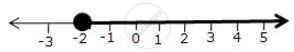Share

Represent the Following Inequalities on Real Number Lines 3x + 1 >= -5 - ICSE Class 10 - Mathematics

ConceptLinear Inequations in One Unknown

Question

Represent the following inequalities on real number lines

3x + 1 >= -5

Solution

3x + 1 ≥ - 5
3x ≥ - 6
x ≥ - 2
Solution on number line isIs there an error in this question or solution?

APPEARS IN

Solution Represent the Following Inequalities on Real Number Lines 3x + 1 >= -5 Concept: Linear Inequations in One Unknown.
S